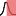# Hypothesis Testing

This category contains 4 nodes.

##### Independent groups t-test

This test is designed to compare the means of observations in the same column between two groups.

##### One-way ANOVA

The one-way analysis of variance (ANOVA) allows to test if any of several means are different from each other.

##### Paired t-test

A paired (or "dependent") t-test is used to compare the means of observations that are not independent of one another.

##### Single sample t-test

The single sample t-test tests the null hypothesis that the population mean is equal to a given number.